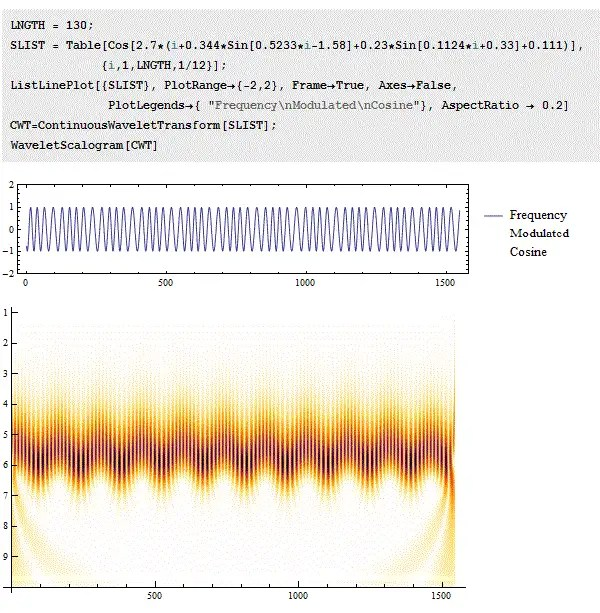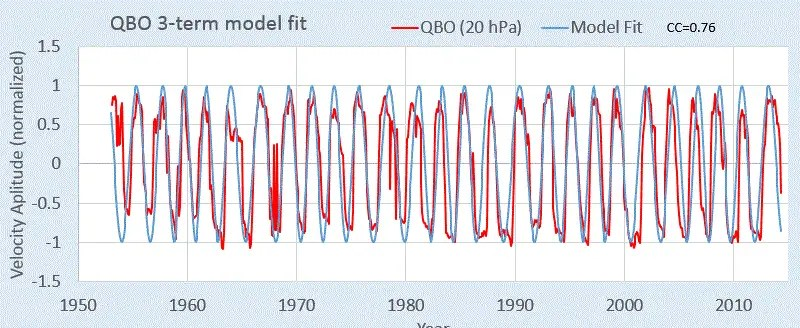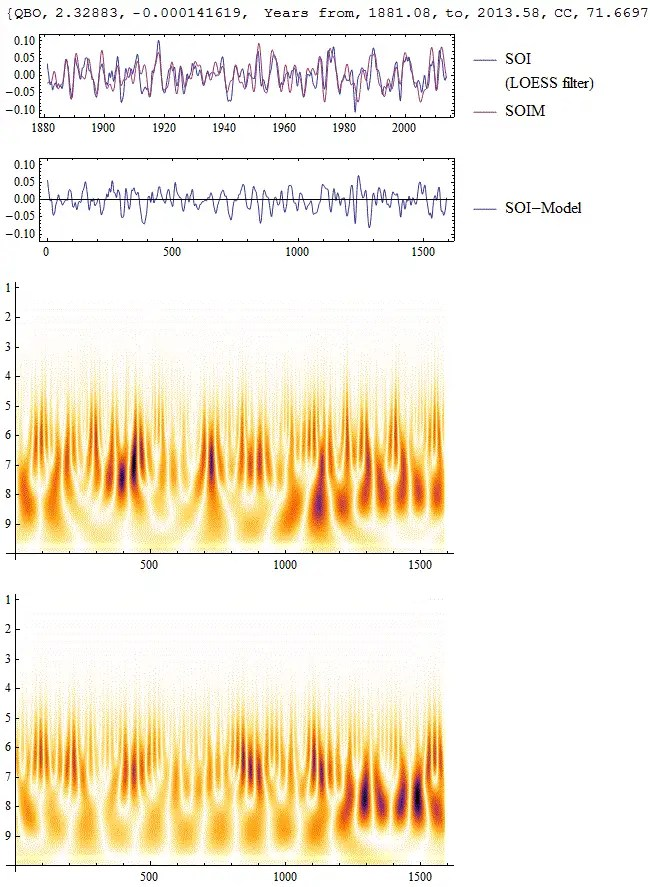# Demodulation and the SOIM

[mathjax]A few people have emailed me asking for a simplified explanation for the model of ENSO that I have been working on. I can appreciate this because presenting solutions to differential equations is not one of the most intuitive ways to convey the essential model.

I decided to go with a mathematical analogy that hopefully will appeal to a techie. Although analogies do not often work when presenting a scientific model, if it turns out that essentially the same mathematical formulation describes the foundation, then it hopefully will serve some use, as many people may already be familiar with the compared-to mathematical construct. So the idea is use the elements of the Southern Oscillation Index Model (SOIM), which includes (1) a basic wave equation, and (2) a forcing provided by stratospheric winds (i.e. the QBO) to convince you that you can understand a model that  effectively describes the seemingly erratic behavior of ENSO. Understanding this concept will be music to your ears if you are willing to listen and appreciate the concept.

In this case the analogy is that of frequency modulation (FM), and specifically what is happening when you listen to an FM station (see Figure 1). Actually, modulation is what occurs during the radio station transmission. But what happens when a radio plays a received FM signal is the process of frequency demodulation. The received broadcast signal is a radio waveform that is modulated not in amplitude but in frequency — and that is what is carrying the music or news over the airwaves. This waveform must be demodulated and then transformed into an amplitude-modulated signal that the human ear can detect.Fig 1:  Example of frequency modulation.  The upper green carrier signal is mixed with a data signal (in red), which becomes the lower frequency in blue.  From here

Electronics ingenuity provides more than one way to demodulate a signal. The simplest conceptual approach relies on a resonant circuit. The carrier frequency is situated near the resonant mode of the circuit, and then the resultant response is detected and amplified in real-time so that the frequency modulation is turned back into an amplitude modulation. The way it does this is by transforming a change in frequency to an amplitude modulation by tracing the slope of the response curve (see Figure 2).Fig 2:   A common way to demodulate is to discriminate the signal by placing the carrier frequency near the edge of the resonant (or characteristic) frequency of the circuit.  A detector can then amplify the demodulated signal. From radio-electronics

The basic resonant circuit in electronics is what is known as an LC-circuit (see inset), consisting of an inductor and a capacitor.The undamped response to a stimulation of such a circuit is mathematically based on solving a second-order differential equation. To set this up formally in terms of a schematic and circuit equations is elementary electrical engineering circuit theory.

$$f”(t) + frac{1}{sqrt{LC}} f(t) = F(t)$$

The square root term is known as the resonant frequency:

$$omega = sqrt{1 over LC}$$

And F(t) is the forcing function, ostensibly the signal sent from the transmitter.

Now we need to consider the ocean and atmospheric analogies to the resonant circuit. The ocean is the receiving resonant circuit, and the atmosphere (specifically, the stratosphere) is the transmitter, via the cyclic wind pattern known as the quasi-biennial oscillation (QBO). Recall that the QBO is called quasi for good reason – not only is the cycle not exactly aligned on 2 year periods, it also is not perfectly periodic.

What we need to watch for is the frequency modulated signal, such as shown in Figure 3 as a mathematically synthesized waveform.Fig 3:  A subtly frequency modulated signal, with the modulation made apparent in the wavelet scalogram shown in the lower panel — the carrier frequency is seen left to right along the time axis, while the frequency modulation appears as a ripple along the vertical axis.

If we take the measure of QBO at altitudes corresponding to atmospheric pressure of 20 hPa (the strongest region for QBO) and plot the waveform, we can see that the modulation is subtle but definitely exists.Fig 4:  The QBO shows a subtle but definite frequency modulation. The blue oscillation is a 3 term sinusoidal approximation to the QBO waveform. This has a “carrier frequency” of approximately 28 months with three embedded sinusoids operating at ~12, 55, and 71 years.

When the QBO acts as a forcing on the Pacific Ocean’s surface via a downward shearing mechanism , the result is the erratic nature of ENSO.  When we solve the basic sloshing model for the ocean’s response, we get a Mathieu (or Hill) second-order differential equation.

$$f”(t) + (a – 2q cos{2t}) f(t) = F(t)$$

Solving this equation for the response f(t) creates essentially the same demodulation that the resonant LC circuit  The SOIM is nothing more than a differential equation solution to the best estimate of the ocean’s response function (LHS=left-hand side) to the QBO and other forcing functions (RHS=right-hand side).Fig 5: One model of the ENSO response as determined by the SOIM. The two lower panels are wavelet scalograms of the data and model.

I walked through this particular model on the Azimuth forum.  The origins of the QBO (see Figure 6) are also discussed on the forum here (scroll down) and here.Fig 6:  The QBO itself may be a resonant response.

That is the basic model and you can see how well it does in capturing the essential erratic nature of ENSO, and showing some real skill in pinpointing the (1) peaks and valleys (2) the multi-frequency phase relationships and (3) the relative amplitudes.

Yet we can also do much better. Looking at one of the forcing and Mathieu/Hill modulation functions that I have been using to fit a solution to SOI, it appeared to have the same periodicity 10-11 years as the total solar irradiance (TSI) variation anomaly.Fig 7 : Total Solar Irradiance as supplied from NASA.

What I tried to do was break up the TSI in two intervals, a varying increasing portion that runs from 1880 to 1980, and then a relatively flat and more periodic interval after 1980. Then I modeled this as two sets of sinusoids that roughly follow this envelope. That is the two if-then conditionals highlighted in yellow in the chart in Figure 8 below (the transition point is indicated as the short red vertical line on the chart). This applied as both a forcing and as a Mathieu modulation to the ocean – if this is a heating modulation then conceivably it changes the characteristics via small density changes.Fig 8 : SOIM solution of the ENSO SOI signal represented by 3 forcing terms (1) QBO , (2) TSI, and (3) a Chandler wobble frequency signal.

The reverse blue highlighted region is the QBO forcing.

Suddenly there are not as many free unattached variables to play around with as I had before. There is one that appears to be connected to the Chandler wobble (~6.4 years) and then a couple of long term 70 and 100 year slow modulations of the characteristic frequency.

It is possible that the basic foundation behind the theory of ENSO has possibly been cracked. And that I can use this as part of a predictive CSALT model for global temperatures. But what this also means is that we have replaced the puzzle regarding the origin of the ENSO SOI behavior with the dual puzzles behind the variation of QBO and TSI / solar variation — the latter of which feature those enigmatic sunspots.

## References

 Gray, William M, John D Sheaffer, and John A Knaff. “Hypothesized Mechanism for Stratospheric QBO Influence on ENSO Variability.” Geophysical Research Letters 19, no. 2 (1992): 107–10.
 ———. “Inﬂuence of the Stratospheric QBO on ENSO Variability.” J. Meteor: Soc. Japan 70 (1992): 975–95.

NEW! The SOIM links page has a comprehensive list of Context/Earth blog posts and Azimuth Forum threads

## 4 thoughts on “Demodulation and the SOIM”

1.dbsetws

są nieodporne zademonstrować sumę uczyń ślubnych, kolektywnie z seksualnymi, skoro stanowią iż
nominowanie się na nie doniesie im jednoznaczną
zaleta. Egzystują szalenie pazerne a jeśliby owszem ostatnie mogę zwerbować – nieugięte.
W 4 wyjątkach na 5 spontanicznie przejęte
zatrzymaniem akcentuj do uzbieranego dobrobycie.

Like